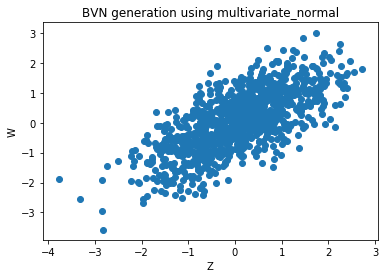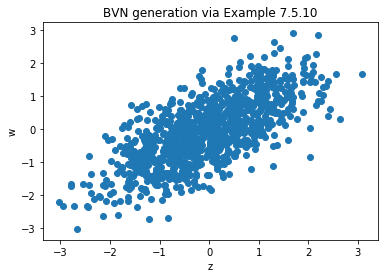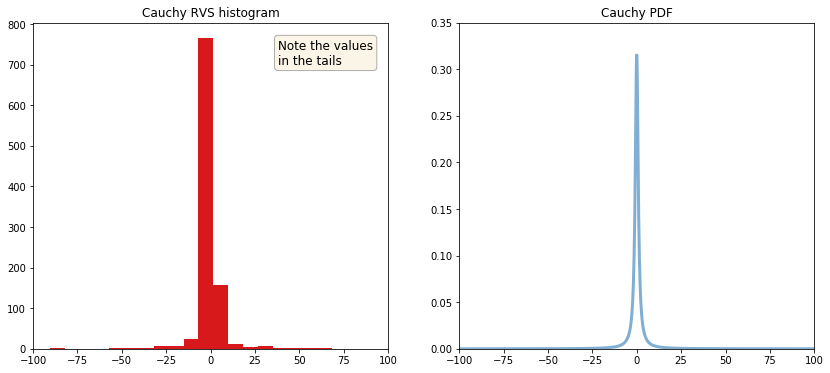# Chapter 7: Joint Distributions¶

This Jupyter notebook is the Python equivalent of the R code in section 7.7 R, pp. 318 - 320, Introduction to Probability, 2nd Edition, Blitzstein & Hwang.

In :
import matplotlib.pyplot as plt
import numpy as np

%matplotlib inline


## Multinomial¶

The functions for the Multinomial distribution represented by scipy.stats.multinomial are pmf (which is the joint PMF of the Multinomial distribution) and rvs (which generates realizations of Multinomial random vectors). The joint CDF of the Multinomial is a pain to work with, so like R it is not built in multinomial.

To use pmf, we have to input the value at which to evaluate the joint PMF, as well as the parameters of the distribution. For example,

In :
from scipy.stats import multinomial

#print(multinomial.__doc__)

In :
x = [2, 0, 3]
n = 5
p = [1/3, 1/3, 1/3]

ans = multinomial.pmf(x, n, p)
print('multinomial.pmf(x, n, p) = {}'.format(ans))

multinomial.pmf(x, n, p) = 0.041152263374485576


returns the probability $P(X_1 = 2, \, X_2 = 0, \, X_3 = 3)$, where

\begin{align} X = (X_1, \, X_2, \, X_3) \sim Mult_3\left(5, \, (\frac{1}{3}, \frac{1}{3}, \frac{1}{3})\right) \end{align}

Of course, n has to equal numpy.sum(x); if we attempted to do multinomial.pmf(x, 7, p), the return value would simply be 0.0.

For rvs, the named function parameter size is the number of Multinomial random vectors to generate, and the other inputs are the same. When we ran rvs(n, p, size=10) with n and p as above, multinomial gave us the following matrix:

In :
# seed the random number generator
np.random.seed(46368)

rv_vector = multinomial.rvs(n, p, size=10)

print('matrix of Multinomial random vectors has shape {}\n'.format(rv_vector.shape))

print(rv_vector)

matrix of Multinomial random vectors has shape (10, 3)

[[2 0 3]
[2 2 1]
[1 1 3]
[3 1 1]
[1 3 1]
[4 0 1]
[0 3 2]
[2 3 0]
[1 2 2]
[3 1 1]]


Each row of the matrix corresponds to a draw from the $Mult_3\left(5, \, (1/3, 1/3, 1/3)\right)$ distribution. In particular, the sum of each row is 5.

## Multivariate Normal¶

Functions for the Multivariate Normal distribution are located in the scipy.stats.multivariate_normal module. As expected, pdf can be used for calculating the joint PDF, and rvs can be used for generating random vectors. For example, suppose that we want to generate 1000 independent Bivariate Normal pairs $(Z, W)$, with correlation $\rho = 0.7$ and $N(0, 1)$ marginals. To do this, we can execute the following:

In :
from scipy.stats import multivariate_normal

#print(multivariate_normal.__doc__)

In :
np.random.seed(75025)

meanvector = [0, 0]
rho = 0.7
covmatrix = np.array([[1, rho],
[rho, 1]])

r = multivariate_normal.rvs(mean=meanvector, cov=covmatrix, size=10**3)
print('matrix r has shape: {}'.format(r.shape))

# take the 1st column of matrix r as Z
Z = r[:, 0]

# take the 2nd column of matrix r as W
W = r[:, 1]

matrix r has shape: (1000, 2)


The covariance matrix here is

\begin{align} \begin{pmatrix} 1 & \rho \\ \rho & 1 \end{pmatrix} \end{align}

because

• $Cov(Z, Z) = Var(Z) = 1$ (this is the upper left entry)
• $Cov(W, W) = Var(W) = 1$ (this is the lower right entry)
• $Cov(Z, W) = Corr(Z, W) \, SD(Z) \, SD(W) = \rho$ (this is the other two entries).

Now r is a 1000 $\times$ 2 matrix, with each row a BVN random vector. To see these as points in the plane, we can use matplotlib.pyplot.scatter(Z, W) to make a scatter plot, from which the strong positive correlation should be clear. To estimate the covariance of Z and W, we can use numpy.cov(Z, W), which computes the true covariance matrix.

In :
plt.scatter(Z, W)

plt.xlabel('Z')
plt.ylabel('W')
plt.title('BVN generation using multivariate_normal')

plt.show()In :
# numpy.cov(x, y) returns a 2 x 2 covariance matrix
#   cov(x,x)    cov(x,y)
#   cov(y,x)    cov(y,y)
est_cov = np.cov(Z, W)
print('covariance matrix for Z and W:\n{}'.format(est_cov))

print('\nestimated covariance of Z and W is {}'.format(est_cov))

covariance matrix for Z and W:
[[0.99653582 0.70792068]
[0.70792068 1.00873687]]

estimated covariance of Z and W is 0.7079206838367835


Example 7.5.10 gives another approach to the BVN generation problem:

In :
np.random.seed(121393)

from scipy.stats import norm

rho = 0.7
tau = np.sqrt(1 - rho**2)
x = norm.rvs(size=10**3)
y = norm.rvs(size=10**3)
z = x
w = rho*x + tau*y


This gives the $Z$-coordinates in an array z and the $W$-coordinates in an array w. If we want to put them into one 1000 $\times$ 2 matrix as we had above, we can use numpy.stack([z, w], axis=1) to bind the arrays together as columns.

In :
# bind z and w into a 1000 x 2 matrix
r2 = np.stack([z, w], axis=1)
print('matrix r2 has shape: {}'.format(r2.shape))

matrix r2 has shape: (1000, 2)


Let's create another scatter plot now with z and w, and also check their estimated covariance.

In :
plt.scatter(z, w)

plt.xlabel('z')
plt.ylabel('w')
plt.title('BVN generation via Example 7.5.10')

plt.show()In :
est_cov2 = np.cov(z, w)
print('covariance matrix for z and w:\n{}'.format(est_cov2))

print('\nestimated covariance of z and w is {}'.format(est_cov2))

covariance matrix for z and w:
[[1.03751418 0.69336422]
[0.69336422 0.94874494]]

estimated covariance of z and w is 0.6933642208178616


## Cauchy¶

We can work with the Cauchy distribution introduced in Example 7.1.24 using the three functions pdf, cdf, and rvs in scipy.stats.cauchy. To shift and/or scale the distribution use the loc and scale parameters. Specifically, cauchy.pdf(x, loc, scale) is identically equivalent to cauchy.pdf(y) / scale with y = (x - loc) / scale.

In :
from scipy.stats import cauchy

#print(cauchy.__doc__)


For an amusing demonstration of the very heavy tails of the Cauchy distribution, try creating a histogram of 1000 simulated values of the Cauchy distribution:

In :
np.random.seed(196418)

fig = plt.figure(figsize=(14, 6))

# create frozen instance of a cauchy distribution
cauch = cauchy()

# generate 1000 random simulated values from cauchy
rv = cauch.rvs(size=1000)

ax1.hist(rv, bins=50, color='#d7191c')
ax1.set_xlim([-100.0, 100.0])
ax1.set_title('Cauchy RVS histogram')
ax1.text(0.69, 0.95,
'Note the values\nin the tails',
transform=ax1.transAxes,
fontsize=12,
verticalalignment='top',
bbox=dict(boxstyle='round', facecolor='wheat', alpha=0.3))

# create a sequqnce of 1000 values from -100 to 100 for x
x = np.linspace(-100, 100, 1000)
# obtain corresponding cauchy PDF values for y
y = cauch.pdf(x)